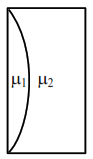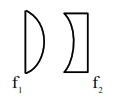# Curved surfaces of a plano

Question:

Curved surfaces of a plano-convex lens of refractive index $\mu_{1}$ and a plano-concave lens of refractive index $\mu_{2}$ have equal radius of curvature as shown in figure. Find the ratio of radius of curvature to the focal length of the combined lenses.1. $\frac{1}{\mu_{2}-\mu_{1}}$

2. $\mu_{1}-\mu_{2}$

3. $\frac{1}{\mu_{1}-\mu_{2}}$

4. $\mu_{2}-\mu_{1}$

Correct Option: , 2

Solution:$\frac{1}{f_{1}}=\left(\mu_{1}-1\right)\left(\frac{1}{R}\right)$

$\frac{1}{f_{2}}=\left(\mu_{2}-1\right)\left(-\frac{1}{R}\right)$

$\frac{1}{\mathrm{f}_{1}}+\frac{1}{\mathrm{f}_{2}}=\frac{1}{\mathrm{f}_{\mathrm{cq}}}=\frac{\left(\mu_{1}-1\right)-\left(\mu_{2}-1\right)}{\mathrm{R}}$

$\frac{1}{\mathrm{f}_{\mathrm{eq}}}=\frac{\left(\mu_{1}-\mu_{2}\right)}{\mathrm{R}}$

$\frac{\mathrm{R}}{\mathrm{f}_{\mathrm{eq}}}=\left(\mu_{1}-\mu_{2}\right)$